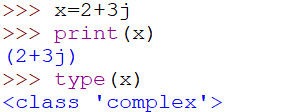top of page• Complex

• # Strings

Float (float)

It is used to represent floating (decimal) point values.

X=10.15

FloatNote: Binary, octal, hexa decimal conventions are not applicable for float.

x=0b10.15    (not applicable)

x=0o10.15  (not applicable)

x=0x10.15    (not applicable)Complex Numbers (complex)

Data type 'complex' is used to represent complex numbers in the form of a+bj, which is is used to perform scientific calculations. To represent imaginary part, only 'j' is allowed in Python; if any other symbol is used, it will give syntax error.

The format is always a+bj, you can not use a+jb.

a = real part, b=imaginary part and j^2=-1

Python 'complex' data type has inbuilt attributes to retrieve real and imaginary parts.

for example x=10+20j

complexif you want to develop mathematical or scientific applications Python is the best choice because it contains Complex data type.a and b can be either int values or float values.Note: in real part if you are using 'int' value you can specify in binary, octal and hexa decimal also. But imaginary part should always be specified in decimal only.Operations on complex numbers:Boolean Values (bool)

It is used to represent Boolean or logical values. Only 2 values are allowed True and False.

Note: T and F must be in capital letter.

boolInternally,  true is treated as 1 and false is treated as 0.

True+True=1+1=2

True+False=1+0=1

True*False=1*0=0

True/False=1/0= ZeroDivisionErrorStrings (str)

string is a sequence of characters.

To represent single line string literal, use '' (single quotes) or "" (double quotes).

strTo represent multi line string literal, use ''' (triple single quotes) or """ (triple double quotes).Slice

Slice mean a piece or substring. In Python, 2 types of indexing are possible means we can access a string from both side: left to right and right to left.

1. +ve index (from left to right)

2. -ve index (from right to left)1. +ve index(from left to right)2. -ve index(from right to left)If we try to access out of range Index.Slice Operator:

Slice Operator is used to divide string into smaller substrings.

S[begin:end]: returns substring from (begin) to (end-1) index.

Ex: s=’knowcubs’

s[1:7]==>nowcub

s[2:7]==>owcubNote 1: end index is optional; if you are not specifying end, default end value is end of the string.

s[1:]==>nowcubsNote 2: begin index is also not mandatory, it is optional; if you are not specifying begin by default begin value is start of the string.

s[:7]==>knowcubNote 3: Even you have an option of not specifying either beginning or end index. In this case output will be full string.Note 4: S[begin:end:step]

By default value of step is step 1.

Ex:   s=’knowcubs’

s[1:8:2]==>nwus

starting from n and ends at s. Here, since step value = 2; so every second character is skipped.Note 5: string repetition operator *

Ex: s=’knowcubs’

s*2=knowscubs knowscubsNote 6: len() is a built-in function in Python. You can use the len() to get the length of the given string, array, list, tuple, dictionary etc.

Ex: s=’knowcubs’For any Query/Suggestion, do let us know in the comment section.

bottom of page